# Bracc.ino - Robotic Arm

Robotic arm controlled by bluetooth controller.

IntermediateWork in progress2,975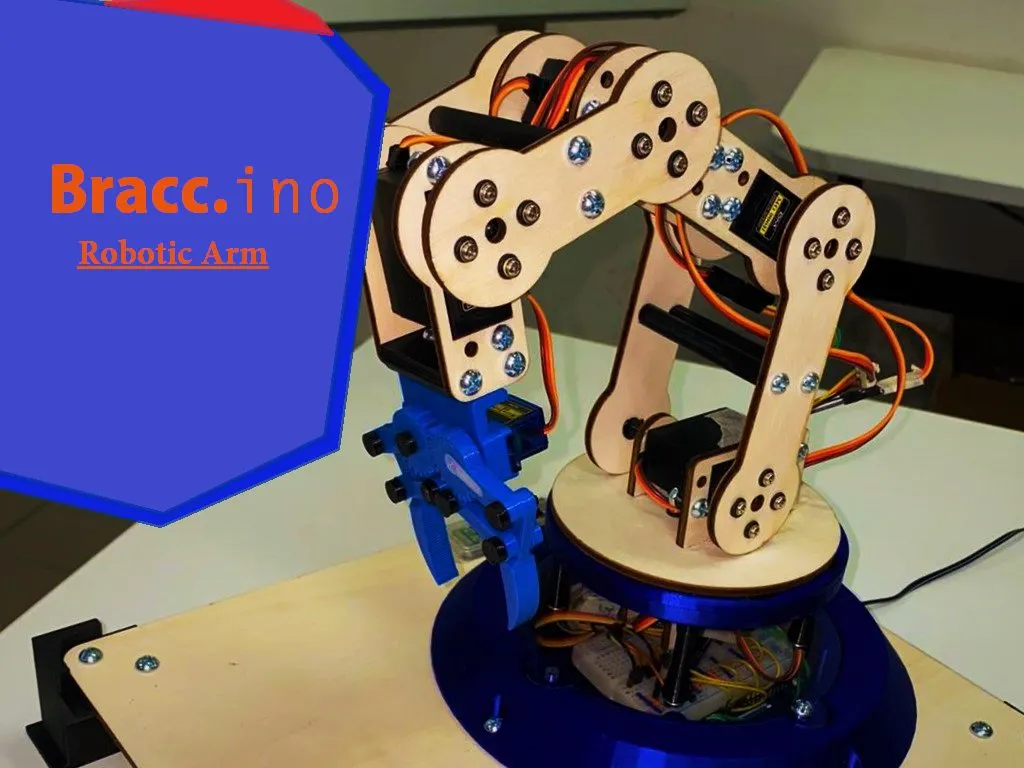## Things used in this project

### Hardware componentsArduino UNO & Genuino UNO
×2×2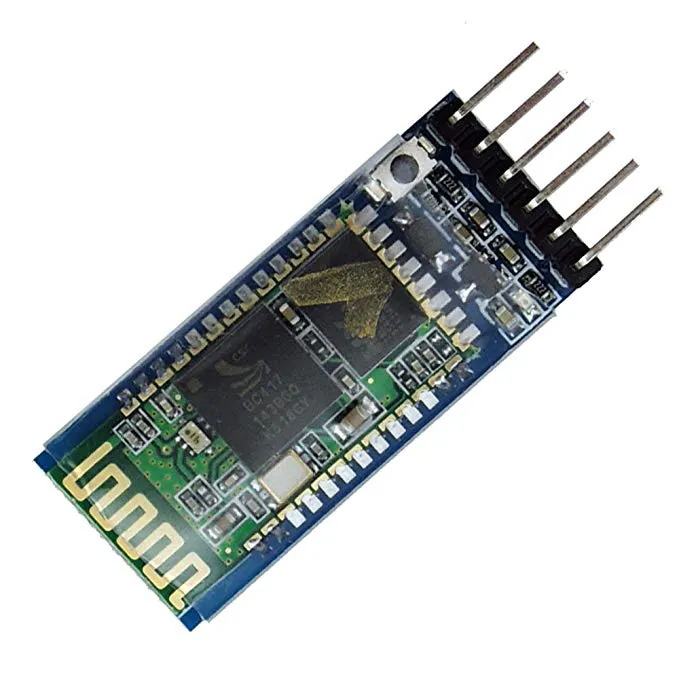HC-05 Bluetooth Module
×2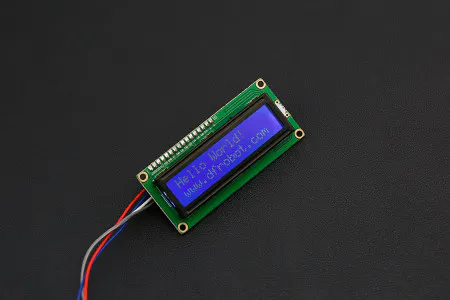DFRobot I2C 16x2 Arduino LCD Display Module
×1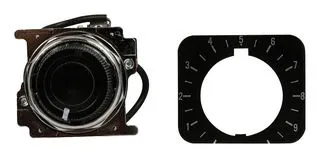Joystick, 10 kohm
×2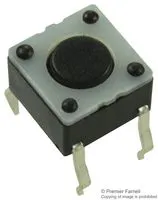Tactile Switch, Top Actuated
×1Resistor 10k ohm
×2
 RESISTOR 2K Ohm
×2Resistor 1k ohm
×2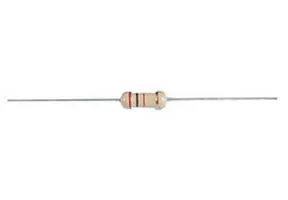Resistor 220 ohm
×2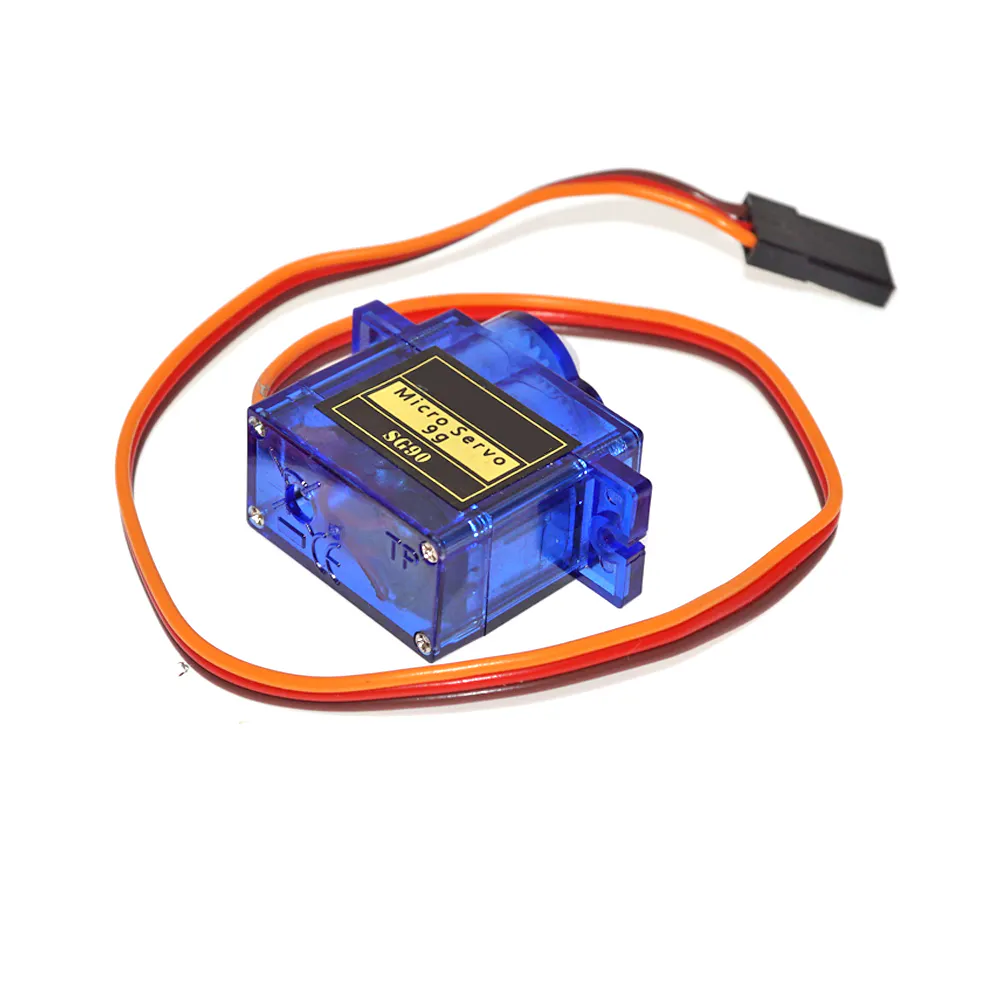SG90 Micro-servo motor
×1
 MG996R Digital Servo
×5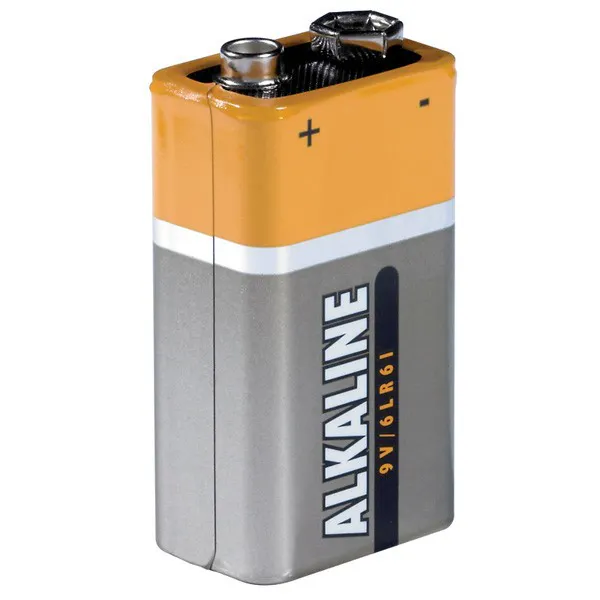9V battery (generic)
×2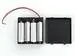4xAA battery holder
×1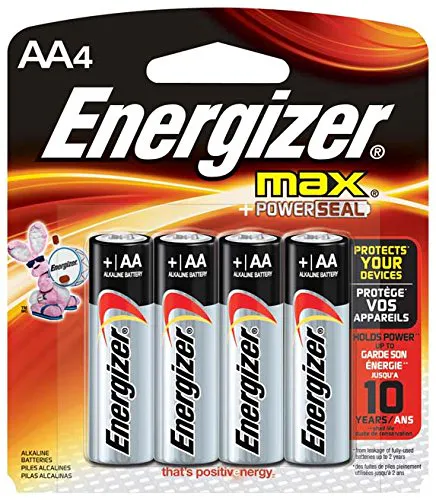AA Batteries
×4Jumper wires (generic)
×1

### Software apps and online servicesArduino IDE

### Hand tools and fabrication machinesHot glue gun (generic)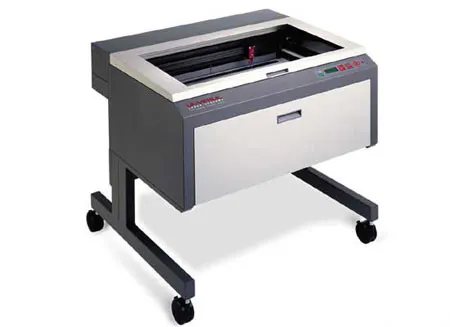Laser cutter (generic)3D Printer (generic)

## Code

### Arm Code

Arduino
```/*
Project: Bracc.ino
Date: 09/01/2020
Last change: 20/01/2020

Team: Basso Andrea
Delmoro Niccol
Gramaglia Giacomo
//////ARM CODE//////
*/

//////////////////
///DECLERATIONS///
//////////////////

/*==LIBRARIES==*/
#include <VarSpeedServo.h>       //Servo library
#include <SoftwareSerial.h>      //Virtual serial communication library, for bluetooth
SoftwareSerial BTserial(0, 1);   //RX (tx hc05) | TX (rx hc05-nodo)

/*==SERVO CONFIGURATIONS==*/
VarSpeedServo base;            //Base Rotation servo
int basePin = 3;
int baseAngle = 90;
const int baseInit = 90;
const int baseMin = 0;
const int baseMax = 180;

VarSpeedServo servo1;          //Shoulder 1 servo
int servo1Pin = 5;                //Pin attached to the servo
int servo1Angle = 73;             //Angle value of the servo
const int servo1Init = 73;        //Initial angle or in HomeMode
const int servo1Min = 0;          //Minimun angle
const int servo1Max = 180;        //Maximun angle

VarSpeedServo servo2;          //Elbow 2 servo
int servo2Pin = 6;
int servo2Angle = 26;
const int servo2Init = 26;
const int servo2Min = 0;
const int servo2Max = 180;

VarSpeedServo servo3;          //Wrist1 3 servo
const int servo3Pin = 9;
int servo3Angle = 147;
const int servo3Init = 147;
const int servo3Min = 0;
const int servo3Max = 180;

VarSpeedServo servo4;          //Wrist2 4 Servo
const int servo4Pin = 10;
int servo4Angle = 14;
const int servo4Init = 14;
const int servo4Min = 0;
const int servo4Max = 180;

VarSpeedServo gripper;        //Gripper servo - SG90
int gripperPin = 11;
int gripperAngle = 60;
int gripperInit = 60;
int gripperMax = 60;
int gripperMin = 0;

/*==BLUETOOTH COMMUNICATION COMMAND==*/
/*==DIRECT MOVEMENT COMMANDS==*/
char MDM = '0';       //Turn on direct motor mode
char MB1 = 'A';       //Move base Clockwise
char MB2 = 'B';       //Move base Counterclockwise
char M11 = 'C';       //Move servo1 Clockwise
char M12 = 'D';       //Move servo1 Counterclockwise
char M21 = 'E';       //Move servo2 Clockwise
char M22 = 'F';       //Move servo2 Counterclockwise
char M31 = 'G';       //Move servo3 Clockwise
char M32 = 'H';       //Move servo3 Counterclockwise
char M41 = 'I';       //Move servo4 Clockwise
char M42 = 'J';       //Move servo4 Counterclockwise
char MG1 = 'K';       //Open gripper
char MG2 = 'L';       //Close gripper
char MHO = 'M';       //Move home point
char MPS = 'N';       //Move points sequence
/*==POINTS COMMAND==*/
char PRD = 'P';       //Reading point
/*==INVERSE KINEMATICS COMMANDS==*/
char CMM = '1';       //Turn in coordinate Mode
char CX1 = 'R';       //Increase X value
char CX2 = 'S';       //Decrease X value
char CY1 = 'T';       //Increase Y value
char CY2 = 'U';       //Decrease Y value
char CZ1 = 'V';       //Increase Z angle
char CZ2 = 'W';       //Decrease Z angle
char CA1 = 'X';       //Grip angle increase-clockwise
char CA2 = 'Y';       //Grip angle decrease-counterclockwise

/*==TIME AND SPEED==*/
int timeUp = 8;               //delay time
int servoSpeed;               //Speed servo general value
int servoSpeedSlow = 15;      //Slow movement speed
int servoSpeedFast = 80;      //Fast movement speed

/*==DIRECT POINTS==*/
int Dpoint1;          //Array for points, each contains servo angles value [base,servo1,servo2,servo3,servo4,gripper]
int Dpoint2;
int Dpoint3;
int Dpoint4;
int Dpoint5;
int DB;                  //Memory for save point
int timeSeq = 2000;      //Time after e sequence

/*==IK VALUES==*/
int XYMode;               //Value for current mode
int x;                    //X cartesian value
int xInit = 95;           //Initial x
int y;                    //Y cartesian value
int yInit = 80;           //Initial y
int gripAngle;            //Gripper angle angle, respect the x axis
int gripAngleInit = 90;   //Initiale angle
int IKpoint1;          //Array for points, each contains coordinate values [x,y,z,angle]
int IKpoint2;
int IKpoint3;
int IKpoint4;
int IKpoint5;
int DBIK;                 //Memory for save point

/*==IK CALCULATION VALUES==*/

//Y offset between base and first joint
int offset = 93;

//1-Translation point G'
int yR;             //Y minus offset
double x2;          //G' x coordinate
double y2;          //G' y coordinate
double angle2;      //Complementar Grip Angle

//2-Slope calculation
double m;           //Pendance of the slope

//3-Circle intersacation
double a;
double b;
double c;
double xB1;
double xB2;
double xB;          //Intersacation x coordinate
double yB;          //Intersacation y coordinate
double r;           //Lenght of line from origin to B point

//4-Triangle angles
double alfa;        //Opposite triangle angle
double beta;        //B point triangle angle
double gamma;       //Origin triangle angle

//5-Deltoid angles
double d;           //Deltoid diagonal
double alfa2;       //Deltoid different angle
double delta;       //Deltoid equal angle value

//6-Angles values
double sum;         //Sum of the first angles
double J1;          //Joint1 Angle
double J2;          //Joint2 Angle
double J3;          //Joint3 Angle
double J4;          //Joint4 Angle

/////////////////
//////SETUP//////
/////////////////

void setup()
{
//Begins
BTserial.begin(38400);
Serial.begin(9600);

//Servo attaching
base.attach(basePin);
servo1.attach(servo1Pin);
servo2.attach(servo2Pin);
servo3.attach(servo3Pin);
servo4.attach(servo4Pin);
gripper.attach(gripperPin);

//Home angle initialitation
moveHome();
}

/////////////////
//////LOOP///////
/////////////////

void loop()
{
limits();
moveAll();
}

/////////////////
/////////////////

{

if (BTserial.available())
{
}
if (reads == MDM)    //DIRECT MODE activation
{
XYMode = 0;
moveHome();
}
else if (reads == CMM)    //INVERSE MODE activation
{
XYMode = 1;
moveHome();

}

if (XYMode == 0)
directMode();
else if (XYMode == 1)
coordinateMode();
}

void limits()           //Limit angle between angleMax and angle Min
{
//Base limitation
if (baseAngle > baseMax)
baseAngle = baseMax;
else if (baseAngle < baseMin)
baseAngle = baseMin;

//Servo1 limitation
if (servo1Angle > servo1Max)
servo1Angle = servo1Max;
else if (servo1Angle < servo1Min)
servo1Angle = servo1Min;

//Servo2 limitation
if (servo2Angle > servo2Max)
servo2Angle = servo2Max;
else if (servo2Angle < servo2Min)
servo2Angle = servo2Min;

//Servo3 limitation
if (servo3Angle > servo3Max)
servo3Angle = servo3Max;
else if (servo3Angle < servo3Min)
servo3Angle = servo3Min;

//Servo4 limitation
if (servo4Angle > servo4Max)
servo4Angle = servo4Max;
else if (servo4Angle < servo4Min)
servo4Angle = servo4Min;

//Gripper limitation
if (gripperAngle > gripperMax)
gripperAngle = gripperMax;
else if (gripperAngle < gripperMin)
gripperAngle = gripperMin;
}

/////////////////
//////MOVES//////
/////////////////

void moveAll()
{
limits();
//Base Movement
base.write(baseAngle, servoSpeed, false);
//Servo1 Movement
servo1.write(servo1Angle, servoSpeed, false);
//Servo2 Movement
servo2.write(servo2Angle, servoSpeed, false);
//Servo3 Movement
servo3.write(servo3Angle, servoSpeed, false);
//Servo4 Movement
servo4.write(servo4Angle, servoSpeed, false);
//Gripper Movement
gripper.write(gripperAngle, servoSpeed, false);
}

void moveHome()
{
servoSpeed = servoSpeedSlow;
if (XYMode == 0)          //Direct Home
{
servoSpeed = servoSpeedSlow;
baseAngle = baseInit;
servo1Angle = servo1Init;
servo2Angle = servo2Init;
servo3Angle = servo3Init;
servo4Angle = servo4Init;
gripperAngle = gripperInit;
}
else if (XYMode == 1)     //Coordinate Home
{
x = xInit;
y = yInit;
gripAngle = gripAngleInit;
baseAngle = baseInit;
IKcalculation();
}
moveAll();
}

/////////////////
//////DKMODE/////
/////////////////

void directMode()
{
if (reads == MB1)        //Base Clockwise
{
baseAngle = baseAngle + 1;
delay(timeUp);
servoSpeed = servoSpeedFast;
}
else if (reads == MB2)   //Base Counterclockwise
{
baseAngle = baseAngle - 1;
delay(timeUp);
servoSpeed = servoSpeedFast;
}

if (reads == M11)        //S1 Clockwise
{
servo1Angle = servo1Angle + 1;
delay(timeUp);
servoSpeed = servoSpeedFast;
}
else if (reads == M12)   //S1 Counterclockwise
{
servo1Angle = servo1Angle - 1;
delay(timeUp);
servoSpeed = servoSpeedFast;
}

if (reads == M21)        //S2 Clockwise
{
servo2Angle = servo2Angle + 1;
delay(timeUp);
servoSpeed = servoSpeedFast;
}
else if (reads == M22)   //S2 Counterclockwise
{
servo2Angle = servo2Angle - 1;
delay(timeUp);
servoSpeed = servoSpeedFast;
}

if (reads == M31)       //S3 Clockwise
{
servo3Angle = servo3Angle + 1;
delay(timeUp);
servoSpeed = servoSpeedFast;
}
else if (reads == M32)  //S3 Counterclockwise
{
servo3Angle = servo3Angle - 1;
delay(timeUp);
servoSpeed = servoSpeedFast;
}

if (reads == M41)       //S4 Clockwise
{
servo4Angle = servo4Angle + 1;
delay(timeUp);
servoSpeed = servoSpeedFast;
}
else if (reads == M42)  //S4 Counterclockwise
{
servo4Angle = servo4Angle - 1;
delay(timeUp);
servoSpeed = servoSpeedFast;
}

{
gripperAngle = 60;
delay(timeUp);
servoSpeed = servoSpeedFast;
}
else if (reads == MG2)  //Closing
{
gripperAngle = 0;
delay(timeUp);
servoSpeed = servoSpeedFast;
}

if (reads == MHO)       //Set angles to home position
{
delay(timeUp);
moveHome();
}

{
savePoint();
}

/*=====Points sequence DIRECT=====*/
{
moveSequence();
}
}

//////////////////POINTS DIRECT///////////////////////////

void   savePoint()
{
if (DB == 0)        //Save point1
{
DB = 1;
}
else if (DB == 1)   //Save point2
{
DB = 2;
}
else if (DB == 2)   //Save point3
{
DB = 3;
}
else if (DB == 3)   //Save point4
{
DB = 4;
}
else if (DB == 4)   //Save point5
{
DB = 5;
}
else if (DB == 5)   //Save Point Home
{
DB = 6;
}
else if (DB == 6)  //Max point allert
{
delay(1000);
}
else if (DB == 7)  //Reset Points
{
DB == 0;
}
}

void moveSequence()
{
servoSpeed = servoSpeedSlow;
if (DB == 6 || DB == 7)          //5 points sequence + Home
{
Point1();
moveAll();
delay(timeSeq);
Point2();
moveAll();
delay(timeSeq);
Point3();
moveAll();
delay(timeSeq);
Point4();
moveAll();
delay(timeSeq);
Point5();
moveAll();
delay(timeSeq);
moveHome();
delay(timeSeq);
}
else if (DB == 5)     //5 points sequence
{
Point1();
moveAll();
delay(timeSeq);
Point2();
moveAll();
delay(timeSeq);
Point3();
moveAll();
delay(timeSeq);
Point4();
moveAll();
delay(timeSeq);
Point5();
moveAll();
delay(timeSeq);
}
else if (DB == 4)                        //4 points sequence
{
Point1();
moveAll();
delay(timeSeq);
Point2();
moveAll();
delay(timeSeq);
Point3();
moveAll();
delay(timeSeq);
Point4();
moveAll();
delay(timeSeq);
}
else if (DB == 3)                        //3 points sequence
{
Point1();
moveAll();
delay(timeSeq);
Point2();
moveAll();
delay(timeSeq);
Point3();
moveAll();
delay(timeSeq);
}
else if (DB == 2)                        //2 points sequence
{
Point1();
moveAll();
delay(timeSeq);
Point2();
moveAll();
delay(timeSeq);
}
else if (DB == 1)                        //1 point sequence
{
Point1();
moveAll();
delay(timeSeq);
}
}

void Point1()
{
baseAngle = Dpoint1;
servo1Angle = Dpoint1;
servo2Angle = Dpoint1;
servo3Angle = Dpoint1;
servo4Angle = Dpoint1;
gripperAngle = Dpoint1;
}
void Point2()
{
baseAngle = Dpoint2;
servo1Angle = Dpoint2;
servo2Angle = Dpoint2;
servo3Angle = Dpoint2;
servo4Angle = Dpoint2;
gripperAngle = Dpoint2;
}
void Point3()
{
baseAngle = Dpoint3;
servo1Angle = Dpoint3;
servo2Angle = Dpoint3;
servo3Angle = Dpoint3;
servo4Angle = Dpoint3;
gripperAngle = Dpoint3;
}
void Point4()
{
baseAngle = Dpoint4;
servo1Angle = Dpoint4;
servo2Angle = Dpoint4;
servo3Angle = Dpoint4;
servo4Angle = Dpoint4;
gripperAngle = Dpoint4;
}
void Point5()
{
baseAngle = Dpoint5;
servo1Angle = Dpoint5;
servo2Angle = Dpoint5;
servo3Angle = Dpoint5;
servo4Angle = Dpoint5;
gripperAngle = Dpoint5;
}

/////////////////
//////IKMODE/////
/////////////////

void coordinateMode()
{
/*=====X VALUE VARIATION=====*/
if (reads == CX1)           //X increase
{
x = x + 1;
delay(timeUp);
servoSpeed = servoSpeedFast;
}
else if (reads == CX2)      //X decrease
{
x = x - 1;
delay(timeUp);
servoSpeed = servoSpeedFast;
}

/*=====Y VALUE VARIATION=====*/
if (reads == CY1)           //Y increase
{
y = y + 1;
delay(timeUp);
servoSpeed = servoSpeedFast;
}
else if (reads == CY2)      //Y decrease
{
y = y - 1;
delay(timeUp);
servoSpeed = servoSpeedFast;
}

/*=====Z ROTATION VARIATION=====*/
if (reads == CZ1)           //Z clockwise rotation
{
baseAngle = baseAngle + 1;
delay(timeUp);
servoSpeed = servoSpeedFast;
}
else if (reads == CZ2)      //Z counterclockwise rotation
{
baseAngle = baseAngle - 1;
delay(timeUp);
servoSpeed = servoSpeedFast;
}

/*=====GRIP ANGLE VARIATION=====*/
if (reads == CA1)           //GripAngle increase
{
gripAngle = gripAngle + 1;
delay(timeUp);
servoSpeed = servoSpeedFast;
}
else if (reads == CA2)      //GripAngle decrease
{
gripAngle = gripAngle - 1;
delay(timeUp);
servoSpeed = servoSpeedFast;
}

/*=====HOME POSITION IK=====*/
{
moveHome();
}

{
gripperAngle = 60;
delay(timeUp);
servoSpeed = servoSpeedFast;
}
else if (reads == MG2)      //Closing
{
gripperAngle = 0;
delay(timeUp);
servoSpeed = servoSpeedFast;
}

{
savePointIK();
}
{
moveSequenceIK();
}
IKcalculation();
}

//////////////////POINTS INVERSE///////////////////////////
void savePointIK()
{
if (DBIK == 0)        //Save point1
{
IKpoint1 = x;
IKpoint1 = y;
IKpoint1 = gripAngle;
DBIK = 1;
}
else if (DBIK == 1)        //Save point2
{
IKpoint1 = x;
IKpoint1 = y;
IKpoint1 = gripAngle;
DBIK = 2;
}
else if (DBIK == 2)        //Save point3
{
IKpoint1 = x;
IKpoint1 = y;
IKpoint1 = gripAngle;
DBIK = 3;
}
else if (DBIK == 3)        //Save point4
{
IKpoint1 = x;
IKpoint1 = y;
IKpoint1 = gripAngle;
DBIK = 4;
}
else if (DBIK == 4)        //Save point5
{
IKpoint1 = x;
IKpoint1 = y;
IKpoint1 = gripAngle;
DBIK = 5;
}
else if (DBIK == 5)        //Save point Home at last
{
DBIK = 6;
}
else if (DBIK == 6)        //Max point allert
{
DBIK = 7;
delay(500);
}
else if (DBIK == 7)        //Reset points
{
DBIK = 0;
delay(1000);
}
}

void moveSequenceIK()
{
servoSpeed = servoSpeedSlow;
if (DBIK == 7 || DBIK == 6)  //Point 1-2-3-4-5-Home Sequence
{
IKPoint1();
delay(timeSeq);
IKPoint2();
delay(timeSeq);
IKPoint3();
delay(timeSeq);
IKPoint4();
delay(timeSeq);
IKPoint5();
delay(timeSeq);
moveHome();
delay(timeSeq);
}
else if (DBIK == 5)          //Point 1-2-3-4-5 Sequence
{
IKPoint1();
delay(timeSeq);
IKPoint2();
delay(timeSeq);
IKPoint3();
delay(timeSeq);
IKPoint4();
delay(timeSeq);
IKPoint5();
delay(timeSeq);
}
else if (DBIK == 4)          //Point 1-2-3-4 Sequence
{
IKPoint1();
delay(timeSeq);
IKPoint2();
delay(timeSeq);
IKPoint3();
delay(timeSeq);
IKPoint4();
delay(timeSeq);
}
else if (DBIK == 3)          //Point 1-2-3 Sequence
{
IKPoint1();
delay(timeSeq);
IKPoint2();
delay(timeSeq);
IKPoint3();
delay(timeSeq);
}
else if (DBIK == 2)          //Point 1-2 Sequence
{
IKPoint1();
delay(timeSeq);
IKPoint2();
delay(timeSeq);
}
else if (DBIK == 1)          //Point 1 Sequence
{
IKPoint1();
delay(timeSeq);
}
}

int Vx;
int Vy;
double pendance;
int Vz;
int Vangle;
double steps;
double q;

void IKPoint1()
{
Vx = IKpoint1 - x;
Vy = IKpoint1 - y;
Vangle = IKpoint1 - gripAngle;
Vz = IKpoint1 - baseAngle;

pendance = Vy / Vx;
q = y - (pendance * x);

if (Vx > 0)
{
for (steps = 0; steps < Vx; steps++)
{
x++;
y = (pendance * x) + q;
if (Vz > 0)
if (baseAngle < IKpoint1)
baseAngle++;
if (Vz < 0)
if (baseAngle > IKpoint1)
baseAngle--;
if (Vangle > 0)
if (gripAngle < IKpoint1)
gripAngle++;
if (Vangle < 0)
if (gripAngle > IKpoint1)
gripAngle--;
delay(100);
IKcalculation();
moveAll();
}
}
if (Vx < 0)
{
for (steps = Vx; steps > 0; steps--)
{
x--;
y = (pendance * x) + q;
if (Vz > 0)
if (baseAngle < IKpoint1)
baseAngle++;
if (Vz < 0)
if (baseAngle > IKpoint1)
baseAngle--;
if (Vangle > 0)
if (gripAngle < IKpoint1)
gripAngle++;
if (Vangle < 0)
if (gripAngle > IKpoint1)
gripAngle--;
delay(100);
IKcalculation();
moveAll();
}
}
}
void IKPoint2()
{
Vx = IKpoint2 - x;
Vy = IKpoint2 - y;
Vangle = IKpoint2 - gripAngle;
Vz = IKpoint2 - baseAngle;

pendance = Vy / Vx;
q = y - (pendance * x);

if (Vx > 0)
{
for (steps = 0; steps < Vx; steps++)
{
x++;
y = (pendance * x) + q;
if (Vz > 0)
if (baseAngle < IKpoint2)
baseAngle++;
if (Vz < 0)
if (baseAngle > IKpoint2)
baseAngle--;
if (Vangle > 0)
if (gripAngle < IKpoint2)
gripAngle++;
if (Vangle < 0)
if (gripAngle > IKpoint2)
gripAngle--;
delay(100);
IKcalculation();
moveAll();
}
}
if (Vx < 0)
{
for (steps = Vx; steps > 0; steps--)
{
x--;
y = (pendance * x) + q;
if (Vz > 0)
if (baseAngle < IKpoint2)
baseAngle++;
if (Vz < 0)
if (baseAngle > IKpoint2)
baseAngle--;
if (Vangle > 0)
if (gripAngle < IKpoint2)
gripAngle++;
if (Vangle < 0)
if (gripAngle > IKpoint2)
gripAngle--;
delay(100);
IKcalculation();
moveAll();
}
}
}
void IKPoint3()
{
Vx = IKpoint3 - x;
Vy = IKpoint3 - y;
Vangle = IKpoint3 - gripAngle;
Vz = IKpoint3 - baseAngle;

pendance = Vy / Vx;
q = y - (pendance * x);

if (Vx > 0)
{
for (steps = 0; steps < Vx; steps++)
{
x++;
y = (pendance * x) + q;
if (Vz > 0)
if (baseAngle < IKpoint3)
baseAngle++;
if (Vz < 0)
if (baseAngle > IKpoint3)
baseAngle--;
if (Vangle > 0)
if (gripAngle < IKpoint3)
gripAngle++;
if (Vangle < 0)
if (gripAngle > IKpoint3)
gripAngle--;
delay(100);
IKcalculation();
moveAll();
}
}
if (Vx < 0)
{
for (steps = Vx; steps > 0; steps--)
{
x--;
y = (pendance * x) + q;
if (Vz > 0)
if (baseAngle < IKpoint3)
baseAngle++;
if (Vz < 0)
if (baseAngle > IKpoint3)
baseAngle--;
if (Vangle > 0)
if (gripAngle < IKpoint3)
gripAngle++;
if (Vangle < 0)
if (gripAngle > IKpoint3)
gripAngle--;
delay(100);
IKcalculation();
moveAll();
}
}
}
void IKPoint4()
{
Vx = IKpoint4 - x;
Vy = IKpoint4 - y;
Vangle = IKpoint4 - gripAngle;
Vz = IKpoint4 - baseAngle;

pendance = Vy / Vx;
q = y - (pendance * x);

if (Vx > 0)
{
for (steps = 0; steps < Vx; steps++)
{
x++;
y = (pendance * x) + q;
if (Vz > 0)
if (baseAngle < IKpoint4)
baseAngle++;
if (Vz < 0)
if (baseAngle > IKpoint4)
baseAngle--;
if (Vangle > 0)
if (gripAngle < IKpoint4)
gripAngle++;
if (Vangle < 0)
if (gripAngle > IKpoint4)
gripAngle--;
delay(100);
IKcalculation();
moveAll();
}
}
if (Vx < 0)
{
for (steps = Vx; steps > 0; steps--)
{
x--;
y = (pendance * x) + q;
if (Vz > 0)
if (baseAngle < IKpoint4)
baseAngle++;
if (Vz < 0)
if (baseAngle > IKpoint4)
baseAngle--;
if (Vangle > 0)
if (gripAngle < IKpoint4)
gripAngle++;
if (Vangle < 0)
if (gripAngle > IKpoint4)
gripAngle--;
delay(100);
IKcalculation();
moveAll();
}
}
}
void IKPoint5()
{
Vx = IKpoint5 - x;
Vy = IKpoint5 - y;
Vangle = IKpoint5 - gripAngle;
Vz = IKpoint5 - baseAngle;

pendance = Vy / Vx;
q = y - (pendance * x);

if (Vx > 0)
{
for (steps = 0; steps < Vx; steps++)
{
x++;
y = (pendance * x) + q;
if (Vz > 0)
if (baseAngle < IKpoint5)
baseAngle++;
if (Vz < 0)
if (baseAngle > IKpoint5)
baseAngle--;
if (Vangle > 0)
if (gripAngle < IKpoint5)
gripAngle++;
if (Vangle < 0)
if (gripAngle > IKpoint5)
gripAngle--;
delay(100);
IKcalculation();
moveAll();
}
}
if (Vx < 0)
{
for (steps = Vx; steps > 0; steps--)
{
x--;
y = (pendance * x) + q;
if (Vz > 0)
if (baseAngle < IKpoint5)
baseAngle++;
if (Vz < 0)
if (baseAngle > IKpoint5)
baseAngle--;
if (Vangle > 0)
if (gripAngle < IKpoint5)
gripAngle++;
if (Vangle < 0)
if (gripAngle > IKpoint5)
gripAngle--;
delay(100);
IKcalculation();
moveAll();
}
}
}

//////////////////
//IK CALCULATION//
//////////////////

void IKcalculation()
{
yR = y - offset;

//1- Translation to G' point
angle2 = 180 - gripAngle;
x2 = x + (link4 * cos(angle2 * (PI / 180.00)));
y2 = yR + (link4 * sin(angle2 * (PI / 180.00)));

//2- Slope calculation
m = y2 / x2;

//3- Circle intersacation
a = 1.00 + sq(m);
b = -(2.00 * x2) - (2.00 * m * y2);
c = sq(x2) + sq(y2) - sq(link3);
xB1 = (-b + sqrt(sq(b) - (4 * a * c))) / (2.00 * a);
xB2 = (-b - sqrt(sq(b) - (4 * a * c))) / (2.00 * a);
xB = min(xB1, xB2);
yB = m * xB;
r = sqrt(sq(xB) + sq(yB));

//4- Triangle angles
beta = (acos((sq(link2) + sq(r) - sq(link1)) / (2.00 * link2 * r))) * (180.00 / PI);
gamma = (acos((sq(link1) + sq(r) - sq(link2)) / (2.00 * link1 * r))) * (180.00 / PI);

//5- Deltoid angles
delta = (180.00 - beta) * (PI / 180.00);
alfa2 = 2.00 * ((asin((link3 * sin(delta)) / d)) * (180.00 / PI));

//6- Joints angles calculation
J1 = gamma + ((atan(y2 / x2)) * (180.00 / PI));
J2 = alfa - 90.00 + alfa2;
J3 = delta * (180.00 / PI) - 90.00;
sum = J1 + alfa + alfa2 + delta * (180 / PI);
J4 = 270 + angle2 - sum;

//7- Servo angles convertion
servo1Angle = 180 - J1;
servo2Angle = J2;
servo3Angle = 180 - J3;
servo4Angle = J4;
}
```

### Joystick Code

Arduino
```/*
Project: Bracc.ino
Date: 09/01/2020
Last change: 23/01/2020

Team: Basso Andrea
Delmoro Niccol
Gramaglia Giacomo
*/
////////////////////
////DECLARATIONS////
////////////////////

/*==LIBRARIES==*/
#include <SoftwareSerial.h>
SoftwareSerial BTserial(2, 3); // HC-05 = 2-RX(+resistors) | 3-TX
#include <LiquidCrystal_I2C.h>
LiquidCrystal_I2C lcd (0x27, 16, 2); //sda=A4 , scl=A5

/*==BUTTONS==*/
int button1Pin = 4;       //Open Gripper
int valButton1;
int button2Pin = 5;       //Save Point
int valButton2;
int button3Pin = 7;       //Close Gripper
int valButton3;
int button4Pin = 8;       //Move sequence
int valButton4;
//Joystick Buttons
int button5Pin = 9;       //Coordinate Mode (NC)
int valButton5;
int button6Pin = 6;       //Home position
int valButton6;

/*==JOYSTICK 1==*/
int joy1xPin = A0;
int joy1yPin = A1;
int valX1;
int valY1;

/*==JOYSTICK 2==*/
int joy2xPin = A2;
int joy2yPin = A3;
int valX2;
int valY2;

/*==ANGLE VARIABLES==*/
//Base
int base = 90;
int baseMax = 180;
int baseMin = 0;
//Servo1
int servo1 = 73;
int servo1Max = 180;
int servo1Min = 0;
//Servo2
int servo2 = 26;
int servo2Max = 180;
int servo2Min = 0;
//Servo3
int servo3 = 147;
int servo3Max = 180;
int servo3Min = 0;
//Servo4
int servo4 = 14;
int servo4Max = 180;
int servo4Min = 0;

/*==Movement Commands==*/
char MDM = '0';       //Turn on direct motor mode
char MB1 = 'A';       //Move base Clockwise
char MB2 = 'B';       //Move base Counterclockwise
char M11 = 'C';       //Move servo1 Clockwise
char M12 = 'D';       //Move servo1 Counterclockwise
char M21 = 'E';       //Move servo2 Clockwise
char M22 = 'F';       //Move servo2 Counterclockwise
char M31 = 'G';       //Move servo3 Clockwise
char M32 = 'H';       //Move servo3 Counterclockwise
char M41 = 'I';       //Move servo4 Clockwise
char M42 = 'J';       //Move servo4 Counterclockwise
char MG1 = 'K';       //Open gripper
char MG2 = 'L';       //Close gripper
char MHO = 'M';       //Move home point
char MPS = 'N';       //Move points sequence
/*==Point Commands==*/
char PRD = 'P';       //Reading point
/*==Coordinate Commands==*/
char CMM = '1';       //Turn in coordinate Mode
char CX1 = 'R';       //Increase X value
char CX2 = 'S';       //Decrease X value
char CY1 = 'T';       //Increase Y value
char CY2 = 'U';       //Decrease Y value
char CZ1 = 'V';       //Increase Z angle
char CZ2 = 'W';       //Decrease Z angle
char CA1 = 'X';       //Grip angle increase-clockwise
char CA2 = 'Y';       //Grip angle decrease-counterclockwise

/*==TIME VALUE==*/
int Speed = 8;

/*==IK VALUES==*/
int XYMode;
int modeTouch;
int x = 95;
int y = 80;
int angle = 90;
int DB;

////////////////////
////////SETUP///////
////////////////////

void setup()
{
//Button declarations
pinMode(button1Pin, INPUT);
pinMode(button2Pin, INPUT);
pinMode(button3Pin, INPUT);
pinMode(button4Pin, INPUT);
pinMode(button5Pin, INPUT);
pinMode(button6Pin, INPUT);

//Joysticks declarations
pinMode(joy1xPin, INPUT);
pinMode(joy1yPin, INPUT);
pinMode(joy2xPin, INPUT);
pinMode(joy2yPin, INPUT);

//Begins
Serial.begin(9600);
BTserial.begin(38400);
lcd.init();
lcd.clear();
lcd.backlight();
lcd.setCursor(0, 0);
lcd.print("Inizialitation...");
}

////////////////////
////////LOOP////////
////////////////////

void loop()
{

//Mode Selection
if (valButton5 == LOW && modeTouch == LOW)   //Enter in IK Mode
{
XYMode = 1;
modeTouch = 1;
delay(Speed);
lcd.clear();
BTserial.write(CMM);
lcd.setCursor(0, 0);
lcd.print("Coordinate XY");
lcd.setCursor(0, 1);
lcd.print("Mode...");
//Initialitation
x = 95;
y = 80;
angle = 90;
base = 90;
delay(1000);
}
else if (valButton5 == LOW && modeTouch == HIGH)  //Exit from IK Mode
{
XYMode = 0;
modeTouch = 0;
delay(Speed);
lcd.clear();
BTserial.write(MDM);
lcd.setCursor(0, 0);
lcd.print("Direct motor");
lcd.setCursor(0, 1);
lcd.print("Mode...");
//Inizialitation
base = 90;
servo1 = 73;
servo2 = 26;
servo3 = 147;
servo4 = 14;
delay(1000);
}

//Mode actions [IK - DIRECT]
if (XYMode == 1)           //IK Mode
{
coordinateMode();
}
else if (XYMode == 0)      //Direct Mode
{
}
}

////////////////////
////////////////////

{

/*===== MG1 - MG2 = GRIPPER =====*/
if (valButton1 == HIGH)             //LowButtonLeft - Gripper opening
{
delay(Speed);
lcd.clear();
BTserial.write(MG1);
lcd.setCursor(0, 0);
lcd.print("Gripper =");
lcd.setCursor(0, 1);
lcd.print("Opened");
}
else if (valButton3 == HIGH)      //LowButtonRight- Gripper Closing
{
delay(Speed);
lcd.clear();
BTserial.write(MG2);
lcd.setCursor(0, 0);
lcd.print("Gripper =");
lcd.setCursor(0, 1);
lcd.print("Closed");
}

/*===== Homing Button =====*/
if (valButton6 == HIGH)            //JoystickRightButton - Active Home
{
delay(Speed);
lcd.clear();
BTserial.write(MHO);
lcd.setCursor(0, 0);
lcd.print("Homing");
base = 90;
servo1 = 73;
servo2 = 26;
servo3 = 147;
servo4 = 14;

x = 95;
y = 80;
angle = 90;
delay(1500);
}

/*===== Points =====*/
if (valButton2 == HIGH)           //HighButtonLeft - Save point position
{
delay(Speed);
lcd.clear();
lcd.setCursor(0, 0);
BTserial.write(PRD);
savePoint();
delay(1500);
}
if (valButton4 == HIGH)           //HighButtonRight - Move points sequence
{
delay(Speed);
lcd.clear();
BTserial.write(MPS);
lcd.setCursor(0, 0);
lcd.print("Move points");
lcd.setCursor(0, 1);
lcd.print("sequence");
delay(1500);
}
}

void limits()  //Limit angle between angleMax and angle Min
{
//base limitation
if (base > baseMax)
base = baseMax;
else if (base < baseMin)
base = baseMin;
//Servo1 limitation
if (servo1 > servo1Max)
servo1 = servo1Max;
else if (servo1 < servo1Min)
servo1 = servo1Min;
//Servo2 limitation
if (servo2 > servo2Max)
servo2 = servo2Max;
else if (servo2 < servo2Min)
servo2 = servo2Min;
//Servo3 limitation
if (servo3 > servo3Max)
servo3 = servo3Max;
else if (servo3 < servo3Min)
servo3 = servo3Min;
//Servo4 limitation
if (servo4 > servo4Max)
servo4 = servo4Max;
else if (servo4 < servo4Min)
servo4 = servo4Min;
}

////////////////////
//////DIRECT////////
////////////////////

{

/*===== MB1 - MB2 = BASE =====*/
if (valX1 > 700 && valX2 > 700)             //joy1-2 SenseLeft - Base Clockwise
{
delay(Speed);
lcd.clear();
BTserial.write(MB1);
base = base + 1;
lcd.setCursor(0, 0);
lcd.print("Base Angle=");
lcd.setCursor(0, 1);
lcd.print(base);
}
else if (valX1 < 350 && valX2 < 350)       //joy1-2 SenseRight - Base Counterclockwise
{
delay(Speed);
lcd.clear();
BTserial.write(MB2);
base = base - 1;
lcd.setCursor(0, 0);
lcd.print("Base Angle=");
lcd.setCursor(0, 1);
lcd.print(base);
}

/*===== M11 - M12 = SERVO 1 =====*/
if (valY1 > 700 && valY2 > 700)            //Joy1-2 SenseDown - Servo1 Clockwise
{
delay(Speed);
lcd.clear();
BTserial.write(M11);
servo1 = servo1 + 1;
lcd.setCursor(0, 0);
lcd.print("Servo1 Angle =");
lcd.setCursor(0, 1);
lcd.print(servo1);
}
else if (valY1 < 350 && valY2 < 350)       //Joy1-2 SenseUp - Servo1 Counterclockwise
{
delay(Speed);
lcd.clear();
BTserial.write(M12);
servo1 = servo1 - 1;
lcd.setCursor(0, 0);
lcd.print("Servo1 Angle =");
lcd.setCursor(0, 1);
lcd.print(servo1);
}

/*===== M21 - M22 = SERVO 2 =====*/
if (valY1 < 350 && valY2 > 400 && valY2 < 600)           //Joy1 SenseDown - Servo2 Clockwise
{
delay(Speed);
lcd.clear();
BTserial.write(M21);
servo2 = servo2 + 1;
lcd.setCursor(0, 0);
lcd.print("Servo2 Angle =");
lcd.setCursor(0, 1);
lcd.print(servo2);
}
else if ( valY1 > 700 && valY2 < 600 && valY2 > 400)       //Joy1 SenseUp - Servo2 Counterclockwise
{
delay(Speed);
lcd.clear();
BTserial.write(M22);
servo2 = servo2 - 1;
lcd.setCursor(0, 0);
lcd.print("Servo2 Angle =");
lcd.setCursor(0, 1);
lcd.print(servo2);
}

/*===== M31 - M32 = SERVO 3 =====*/
else if (valY2 > 700 && valY1 > 400 && valY1 < 600)             //Joy2 SenseDown - Servo3 Clockwise
{
delay(Speed);
lcd.clear();
BTserial.write(M31);
servo3 = servo3 + 1;
lcd.setCursor(0, 0);
lcd.print("Servo3 Angle =");
lcd.setCursor(0, 1);
lcd.print(servo3);
}
else if (valY2 < 350 && valY1 > 400 && valY1 < 600)       //Joy2 SenseUp - Servo3 Counterclockwise
{
delay(Speed);
lcd.clear();
BTserial.write(M32);
servo3 = servo3 - 1;
lcd.setCursor(0, 0);
lcd.print("Servo3 Angle =");
lcd.setCursor(0, 1);
lcd.print(servo3);
}

/*===== M41 - M42 = SERVO 4 =====*/
if (valX1 > 700 && valX2 < 350)             //Joy1 SenseLeft 2 SenseRight - Servo4 Clockwise
{
delay(Speed);
lcd.clear();
BTserial.write(M41);
servo4 = servo4 + 1;
lcd.setCursor(0, 0);
lcd.print("Servo4 Angle =");
lcd.setCursor(0, 1);
lcd.print(servo4);
}
else if (valX1 < 350 && valX2 > 700)      //Joy1 SenseRight 2 SenseLeft - Servo4 Counterclockwise
{
delay(Speed);
lcd.clear();
BTserial.write(M42);
servo4 = servo4 - 1;
lcd.setCursor(0, 0);
lcd.print("Servo4 Angle =");
lcd.setCursor(0, 1);
lcd.print(servo4);
}
limits();
}

////////////////////
//////INVERSE///////
////////////////////

void coordinateMode()
{

/*==X VARIATION - JOYSTICK1 RIGHT/LEFT==*/
if (valX1 > 700)            //X Increase - right
{
delay(Speed);
BTserial.write(CX1);
x = x + 1;
lcd.clear();
}
else if (valX1 < 350)       //X Decrease - left
{
delay(Speed);
BTserial.write(CX2);
x = x - 1;
lcd.clear();
}

/*==Y VARIATION - JOYSTICK1 UP/DOWN==*/
if (valY1 > 700)            //Y increase - Up
{
delay(Speed);
BTserial.write(CY1);
y = y + 1;
lcd.clear();
}
else if (valY1 < 350)       //Y Decrease - Down
{
delay(Speed);
BTserial.write(CY2);
y = y - 1;
lcd.clear();
}

/*==Z BASE ROTATION==*/
if (valX2 > 700)            //Base rotation clockwise
{
delay(Speed);
BTserial.write(CZ1);
base = base + 1;
lcd.clear();
}
else if (valX2 < 350)       //Base rotation counterclockwise
{
delay(Speed);
BTserial.write(CZ2);
base = base - 1;
lcd.clear();
}

/*==GRIP ANGLE VARIATION==*/
if (valY2 > 700)            //Grip clockwise (+)
{
delay(Speed);
BTserial.write(CA1);
angle = angle - 1;
lcd.clear();
}
else if (valY2 < 350)       //Grip counterclockwise (-)
{
delay(Speed);
BTserial.write(CA2);
angle = angle - 1;
lcd.clear();
}

lcd.setCursor(0, 0);
lcd.print("X");
lcd.setCursor(1, 0);
lcd.print(x);
lcd.setCursor(6, 0);
lcd.print("Y");
lcd.setCursor(7, 0);
lcd.print(y);
lcd.setCursor(12, 0);
lcd.print("Z=");
lcd.setCursor(13, 0);
lcd.print(base);
lcd.setCursor(0, 1);
lcd.print("GripAngle=");
lcd.setCursor(10, 1);
lcd.print(angle);
}

////////////////////
////POINT SAVE//////
////////////////////

void savePoint()
{
if (DB == 0)      //Save point1
{
lcd.print("Saved point 1");
DB = 1;
}
else if (DB == 1) //Save point2
{
lcd.print("Saved point 2");
DB = 2;
}
else if (DB == 2) //Save point3
{
lcd.print("Saved point 3");
DB = 3;
}
else if (DB == 3) //Save point4
{
lcd.print("Saved point 4");
DB = 4;
}
else if (DB == 4) //Save point5
{
lcd.print("Saved point 5");
DB = 5;
}
else if (DB == 5) //Save point Home
{
lcd.print("Last Point");
lcd.setCursor(0, 1);
lcd.print("= Home");
DB = 6;
}
else if (DB == 6) //Max point allarm
{
lcd.print("MAX Points!!");
lcd.setCursor(0, 1);
lcd.print("click for reset...");
DB = 7;
}
else if (DB == 7) //Reset poits memories
{
lcd.print("Reset points");
lcd.setCursor(0, 1);
lcd.print("Memories");
DB = 0;
}
}
```

## Credits

### DelmoroN

1 project • 1 follower

### BassoAndrea02

1 project • 1 follower

### momograma

2 projects • 1 follower